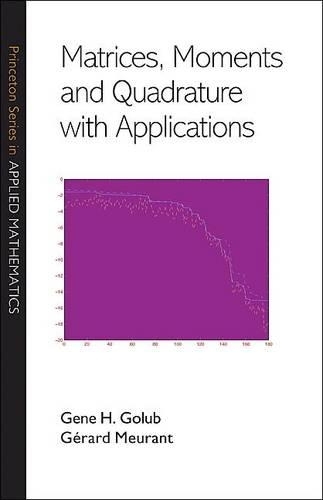Some orders may be delayed by the current Coronavirus lockdown.•# Matrices, Moments and Quadrature with Applications - Princeton Series in Applied Mathematics (Hardback)

(author), (author)
£74.00
Hardback 376 Pages / Published: 22/01/2010
• We can order this

Usually dispatched within 3 weeks

This computationally oriented book describes and explains the mathematical relationships among matrices, moments, orthogonal polynomials, quadrature rules, and the Lanczos and conjugate gradient algorithms. The book bridges different mathematical areas to obtain algorithms to estimate bilinear forms involving two vectors and a function of the matrix. The first part of the book provides the necessary mathematical background and explains the theory. The second part describes the applications and gives numerical examples of the algorithms and techniques developed in the first part. Applications addressed in the book include computing elements of functions of matrices; obtaining estimates of the error norm in iterative methods for solving linear systems and computing parameters in least squares and total least squares; and solving ill-posed problems using Tikhonov regularization. This book will interest researchers in numerical linear algebra and matrix computations, as well as scientists and engineers working on problems involving computation of bilinear forms.

Publisher: Princeton University Press
ISBN: 9780691143415
Number of pages: 376
Weight: 652 g
Dimensions: 235 x 152 x 28 mm